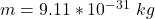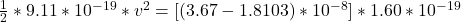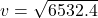## Two stationary positive point charges, charge 1 of magnitude 3.25 nC and charge 2 of magnitude 2.00 nC , are separated by a distance of 58.0

Question

Two stationary positive point charges, charge 1 of magnitude 3.25 nC and charge 2 of magnitude 2.00 nC , are separated by a distance of 58.0 cm . An electron is released from rest at the point midway between the two charges, and it moves along the line connecting the two charges.

Required:

What is the speed of the electron when it is 10.0 cm from the +3.00-nC charge?

in progress 0
6 months 2021-08-09T06:57:21+00:00 1 Answers 0 views 0

1. Complete Question

Two stationary positive point charges, charge 1 of magnitude 3.25 nC and charge 2 of magnitude 2.00 nC , are separated by a distance of 58.0 cm . An electron is released from rest at the point midway between the two charges, and it moves along the line connecting the two charges.

Required:

What is the speed of the electron when it is 10.0 cm from the +3.25-nC charge?

The velocity is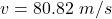Explanation:

From  the question we are told that

The magnitude of charge one is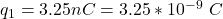The  magnitude of charge twoThe distance of separation is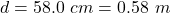Generally the electric potential of the electron at the midway point is mathematically represented as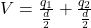substituting values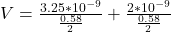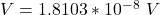Now when the electron is 10 cm   =  0.10 m  from charge 1 , it is  (0.58 – 0.10 =  0.48 m ) m from charge two

Now the electric potential  at that point is mathematically represented as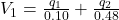substituting values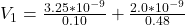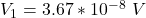Now the law of energy conservation ,

The  kinetic energy of the electron  =  potential energy of the electron

i.e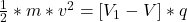where q is the magnitude of the charge on the electron with value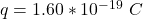While m is the mass of the electron with value